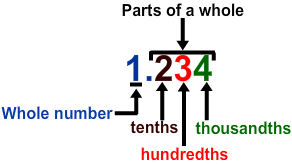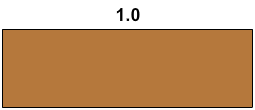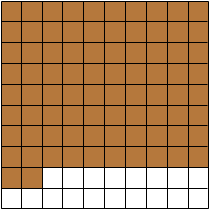# Decimals to Fractions

Print Rate 0 stars Common Core
Lesson size:
Message preview:
Someone you know has shared lesson with you:

To play this lesson, click on the link below:

https://www.turtlediary.com/lesson/decimals-to-fractions.html

Hope you have a good experience with this site and recommend to your friends too.

Login to rate activities and track progress.
Login to rate activities and track progress.

We know that decimals and fractions both are ways of showing parts of a whole. With decimals, the parts are always broken into tenths, hundredths, thousandths, and so on. The digits to the left of the decimal point represent a whole number. The digits to the right of the number represent the parts of a whole.

When we use decimals, we show all the digits horizontally or in a line.Suppose there is one whole pan of brownies. It would be the same as the digit 1 to the left of the decimal point. Since there are no parts of a whole brownie, there would be a zero to the right of the decimal point. One whole is the same as 1.0A second pan of brownies that had been cut into equal parts, but some pieces had been eaten, is like the digits to the right of the decimal point.

If 4 pieces out of 10 had been eaten (and since 10 - 4 = 6), then we would say 0.6 or 6 tenths of the brownie remains. We just count the tenths!Now, if we want to convert this to a fraction, we just show the parts of the whole.

So, 0.6 =
6
106 out of 10 pieces of brownie

Everything is not always cut into 10 equal parts like the brownie. What if something was cut into one hundred parts?

Well, then we would move two digits to the right of the decimal point.

So, if we had a really large pan of brownies cut into 100 parts and 18 pieces were eaten (100 - 18 = 82), then 0.82 or eighty-two hundredths are the parts of the whole brownie that remain.We can write 0.82 in fraction form as
82
100
.

Here's a strategy to help in converting decimals to fractions.

• If the decimal is in the tenths, just write the digits in decimal as the numerator over a denominator of 10.
• If the decimal is in the hundredths, write the digits in decimal as the numerator over a denominator of 100.
• If the decimal is in the thousandths, put the digits in decimal as the numerator over a denominator of 1000.

Remember to reduce the obtained fraction into its lowest form.

Let's take a look at some examples.

## Example 1

Write 0.7 as a fraction.

0.7 =
7
10

## Example 2

Write 0.75 as a fraction.

0.75 =
75
100

Now, reduce
75
100
into its lowest form.

75
100
=
75 ÷ 25
100 ÷ 25
=
3
4

So, 0.75 =
3
4

## Example 3

Write 0.126 as a fraction.

0.126 =
126
1000

Now, reduce
126
1000
into its lowest form.

126
1000
=
126 ÷ 2
1000 ÷ 2
=
63
500

So, 0.126 =
63
500

## Decimals to Fractions

• Decimals and fractions both are ways of showing parts of a whole.
• To convert a decimal to a fraction, follow these steps:
• Write the digits in the decimal as the numerator.
• Write as many zeroes after 1 in the denominator, as there were decimal places (digits to the right of the decimal point) in the decimal number.
• Reduce the fraction to its lowest form.

## Similar Lessons

Become premium member to get unlimited access.